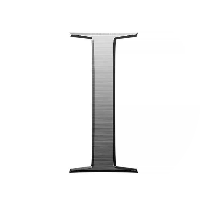# Basic Structures: Sets, Functions, Sequences, Sums and Matrices

This quiz contains multiple-choice problems on sets and their operations and types, Venn diagram, subsets, functions and its growth, algebraic laws, range and domain of functions, arithmetic and geometric sequences, special and harmonic sequences, matrices types, properties and operations, transpose and inverse of matrices, sequences and summations.

Start Quiz

{x: x is a real number between 1 and 2} is a/an

Infinite set

Finite set

Empty set

None of the above

{x: x is an integer that is neither positive nor negative} is a/an

Empty set

Non-empty set

Finite set

Non-empty and finite set

Which of the following expresses the set {1, 5, 15, 25,…} in set-builder form.

{x: either x=1 or x=5n, where n is a real number}

{x: either x=1 or x=5n, where n is a integer}

{x: either x=1 or x=5n, where n is an odd natural number}

{x: x=5n, where n is a natural number}

Which of the following expresses {x: x= n/ (n+1), n is a natural number less than 7} in roster form?

{1⁄2, 2⁄3, 4⁄5, 6⁄7}

{1⁄2, 2⁄3, 3⁄4, 4⁄5, 5⁄6, 6⁄7, 7⁄8}

{1⁄2, 2⁄3, 3⁄4, 4⁄5, 5⁄6, 6⁄7}

Infinite set

Which of the following is a subset of the set {1, 2, 3, 4}?

{1, 2}

{1, 2, 3}

{1}

All of the above

What is the total number of elements in the power set of {a, b}, where a and b are distinct elements?

3

4

2

5

Which of the following is true for A = {∅, {∅}, 2, {2, ∅}, 3}?

{{∅,{∅}} ∈ A

{2} ∈ A

∅ ⊂ A

3 ⊂ A

Which of the following is a subset of the set A = { }?

A

{}

All of the above

Which of the following expresses {x: x is a positive prime number which divides 72} in roster form?

{2, 3, 5}

{2, 3, 6}

{2, 3}

{∅}

The union of the sets {1, 2, 5} and {1, 2, 6} is the set

{1, 2, 6, 1}

{1, 2, 5, 6}

{1, 2, 1, 2}

{1, 5, 6, 3}

The intersection of the sets {1, 2, 5} and {1, 2, 6} is the set

{1, 2}

{5, 6}

{2, 5}

{1, 6}

Two sets are called disjoint if their __ results in an empty set.

Union

Difference

Intersection

Complement

{x: x ∈ N and x is prime} is a/an

Infinite set

Finite set

Empty set

Not a set

The difference of {1, 2, 3} and {1, 2, 5} is the set

{1}

{5}

{3}

{2}

Which of the following set pairs is disjoint?

{1, 3, 5} and {1, 3, 6}

{1, 2, 3} and {1, 2, 3}

{1, 3, 5} and {2, 3, 4}

{1, 3, 5} and {2, 4, 6}

Quiz/Test Summary
Title: Basic Structures: Sets, Functions, Sequences, Sums and Matrices
Questions: 15
Contributed by: